# Evaporation Coefficient (Ke)

The MABIA method adopts the ‘dual’ Kc method, where the Kc value is divided into a ‘basal’ crop coefficient, Kcb, and a separate component, Ke, representing evaporation from the soil surface that is not under the crop canopy.

When the soil surface layer is wet, following rain or irrigation, Ke is at some maximum rate where Kcb + Ke is limited by a maximum value Kc max, and when the soil surface layer is dry, Ke is small and can approach zero.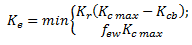where

Kc max = the maximum value of Kc following rain or irrigation,
Kr = a dimensionless evaporation reduction coefficient (defined later) and is dependent on the cumulative depth of water depleted (evaporated), and
few = the fraction of the soil that is both exposed to solar radiation and that is wetted.

The evaporation rate is restricted by the estimated amount of energy available at the exposed soil fraction, i.e., Ke cannot exceed few Kc max

The calculation procedure consists in determining:

• The upper limit Kc max;

• The soil evaporation reduction coefficient Kr; and

• The exposed and wetted soil fraction few.

## Upper limit (Kc max)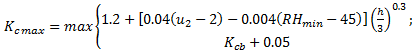where

u2 = wind speed measured at 2 m height [m/s],
RHmin = minimum relative humidity [%],
h = plant height during the current day [m]

This Equation ensures that Kc max is always greater than or equal to the sum Kcb + 0.05, suggesting that wet soil increases the Kc value above Kcb by 0.05 following complete wetting of the soil surface, even during periods of full ground cover.

## Soil evaporation reduction coefficient (Kr)

Soil evaporation from the exposed soil can be assumed to take place in two stages: an energy limiting stage, and a falling rate stage. When the soil surface is wet, Kr is 1. When the water content in the upper soil becomes limiting, Kr decreases.  Kr becomes zero when the total amount of water that can be evaporated (TEW) from the topsoil is depleted.  Readily evaporable water (REW) is the water content that can be evaporated in the first stage (energy limiting).

The amount of water that can be removed by evaporation during a complete drying cycle is estimated as:

TEW = 10 (qFC - 0.5 qWP ) Ze

where

TEW = total evaporable water, the maximum depth of water that can be evaporated from the surface soil layer assuming that the soil was completely wetted [mm],
q
FC = field capacity [% vol]
q
WP = wilt point [% vol]
Ze = the effective depth of the surface soil subject to drying to 0.5 qWP by way of evaporation [m].

Ze is an empirical value based on observation. Some evaporation or soil drying will be observed to occur below the Ze depth. A fixed value of 0.08 m is used in the MABIA method.

The readily evaporable water (REW), which is the maximum depth of water that can be evaporated from the topsoil layer without restriction, is defined as:

REW=(3.121 TEW + 22.896) Ze

The Kr coefficient is calculated as: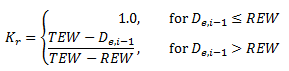where

De,i-1 = cumulative depletion from the soil surface layer at the end of day i-1 (the previous day) [mm],
TEW = total evaporable water [mm],
REW = readily evaporable water [mm]

Evaporation from the soil beneath the crop canopy, occurring at a slower rate, is assumed included in the basal Kcb coefficient.

## Exposed and wetted soil fraction (few)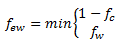where

fw = fraction of the surface wetted by irrigation and/or precipitation,
fc = fraction of soil surface effectively covered by vegetation

fw defines the potential spatial extent of evaporation. Common values for fw are listed in lookup tables. When the soil surface is completely wetted, as by precipitation or sprinkler irrigation, few is set equal to (1 - fc). For irrigation systems where only a fraction of the ground surface (fw) is wetted, few is limited to fw.  Both (1 -fc) and fw, for numerical stability, have limits of [0.01-1]. In the case of drip irrigation, Allen et al. (1998) suggest that where the majority of soil wetted by irrigation is beneath the crop canopy and is shaded, fw be reduced to about one-half to one-third of that given in lookup tables. Their general recommendation for drip irrigation is to multiply fw by [1 - (2/3) fc].

## Soil fraction covered by vegetation (fc)

The value for fc ranges between 0 and 0.99 for numerical stability and is generally determined by visual observation. For purposes of estimating few, fc can be estimated from Kcb as: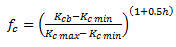where

Kc min = the minimum Kc for dry bare soil with no ground cover,
h = plant height during the current day [m].

The difference Kcb - Kc min is limited to >=0.01 for numerical stability. The value for fc will change daily as Kcb changes. Kc min ordinarily has the same value as Kcb ini used for annual crops under nearly bare soil conditions (i.e., Kc min = 0.15). However, Kc min is set to 0 or nearly zero under conditions with large time periods between wetting events, for example in applications with natural vegetation in deserts. The value for fc decreases during the late season period in proportion to Kcb to account for local transport of sensible heat from senescing leaves to the soil surface.

## Fraction Wetted (fw)

If the most recent wetting event is irrigation, fw = fw irr, which is entered as data.  If the most recent wetting event is precipitation, fw = 1.

## Water Balance of the Soil Surface Layer

Estimation of Ke requires a daily water balance for the few fraction of the surface soil layer. The daily soil water balance equation is:where

De,i-1 and De,i  = cumulative depletion depth at the ends of days i-1 and i [mm],
Pi and ROi = precipitation and precipitation runoff from the soil surface on day i [mm],
Ii = the irrigation depth on day i that infiltrates the soil [mm],
Ei = evaporation on day i (i.e., Ei = Ke ETo) [mm],
Te, i = the depth of transpiration from the exposed and wetted fraction of the soil surface layer on day i [mm],
DPe, i = the deep percolation from the few fraction of the soil surface layer on day i if soil water content exceeds field capacity [mm] to the lower part of the top bucket.

Assuming that the surface layer is at field capacity following heavy rain or irrigation, the minimum value for De,i is zero. The limits imposed on De,i are consequently 0 <= De,i <= TEW. It is recognized that water content of the soil surface layer can exceed TEW for short periods of time while drainage is occurring. However, because the length of time that this occurs varies with soil texture, wetting depth, and tillage, De,i >= 0 is assumed. The irrigation depth Ii is divided by fw to approximate the infiltration depth to the fw portion of the soil surface. Similarly, Ei is divided by few because it is assumed that all Ei (other than residual evaporation implicit to the Kcb coefficient) is taken from the few fraction of the surface layer.  The amount of transpiration extracted from the few fraction of the evaporating soil layer is generally a small fraction of total transpiration, and is generally ignored (Te,i = 0).  DPe,i is not to be confused with deep percolation from the root zone to the second bucket .

## Effective Precipitation

Many factors influence how much precipitation can infiltrate the soil and be available for evapotranspiration (so-called "effective" precipitation), including rainfall rate, terrain slope, and soil type and compaction.  Any precipitation which is not available for ET will become surface runoff.

ROi = Min( MIR, Pi (1 - EP) )

where

MIR  = maximum infiltration rate -- water depth that can infiltrate over a 24 hour period [mm/day],
EP  = effective precipitation rate [%],
P
i and ROi = precipitation and precipitation runoff from the soil surface on day i [mm],